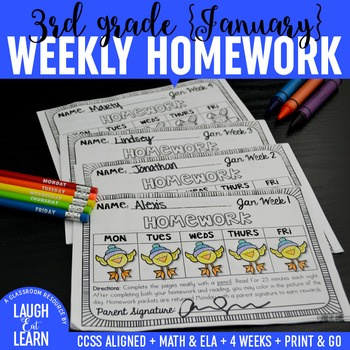# Third Grade Math & ELA Homework: January

Rated 5 out of 5, based on 28 reviews
28 Ratings;
3rd
Subjects
Resource Type
Standards
Formats Included
• PDF
Pages
25 pages
Report this resource to TPT
##### Also included in
1. Students (and teachers!) will love these simple weekly homework packets! These easy to use homework packets can continue to practice their Math and ELA at home with their families in a quick and simple manner. Homework packets are made with a teacher in mind: standards on each page, two per page mak
Price \$16.20Original Price \$18.00Save \$1.80
2. Students (and teachers!) will love these simple weekly homework packets! These easy to use homework packets can continue to practice their Math and ELA at home with their families in a quick and simple manner. Homework packets are made with a teacher in mind: standards on each page, two per page mak
Price \$59.40Original Price \$66.00Save \$6.60

### Description

Students (and teachers!) will love these simple weekly homework packets! These easy to use homework packets can continue to practice their Math and ELA at home with their families in a quick and simple manner. Homework packets are made with a teacher in mind: standards on each page, two per page making it VERY easy to copy, staple and cut in half, and are completely aligned with common core and can be interchangeable throughout the other months I've created for Third Graders.

Included

• Weekly Homework Covers

• 12 Math Homework Sheets

• 8 ELA Homework Sheets

You May Also Like

All ideas are mine unless otherwise stated. Please note that this product is for one teacher/classroom only and not to be shared in any matter. Additional licenses can be purchased through my TPT store at a discounted rate. If there are any questions, comments or changes you need feel free to email me at laugheatlearn@gmail.com and don't forget to review!

You can also connect with me and find many activities

and strategies about homework over at my blog Laugh Eat Learn.

Total Pages
25 pages
N/A
Teaching Duration
N/A
Report this resource to TPT
Reported resources will be reviewed by our team. Report this resource to let us know if this resource violates TPT’s content guidelines.

### Standards

to see state-specific standards (only available in the US).
Determine the unknown whole number in a multiplication or division equation relating three whole numbers. For example, determine the unknown number that makes the equation true in each of the equations 8 × ? = 48, 5 = __ ÷ 3, 6 × 6 = ?.
Apply properties of operations as strategies to multiply and divide. Examples: If 6 × 4 = 24 is known, then 4 × 6 = 24 is also known. (Commutative property of multiplication.) 3 × 5 × 2 can be found by 3 × 5 = 15, then 15 × 2 = 30, or by 5 × 2 = 10, then 3 × 10 = 30. (Associative property of multiplication.) Knowing that 8 × 5 = 40 and 8 × 2 = 16, one can find 8 × 7 as 8 × (5 + 2) = (8 × 5) + (8 × 2) = 40 + 16 = 56. (Distributive property.)
Understand division as an unknown-factor problem. For example, find 32 ÷ 8 by finding the number that makes 32 when multiplied by 8.
Fluently multiply and divide within 100, using strategies such as the relationship between multiplication and division (e.g., knowing that 8 × 5 = 40, one knows 40 ÷ 5 = 8) or properties of operations. By the end of Grade 3, know from memory all products of two one-digit numbers.
Identify arithmetic patterns (including patterns in the addition table or multiplication table), and explain them using properties of operations. For example, observe that 4 times a number is always even, and explain why 4 times a number can be decomposed into two equal addends.